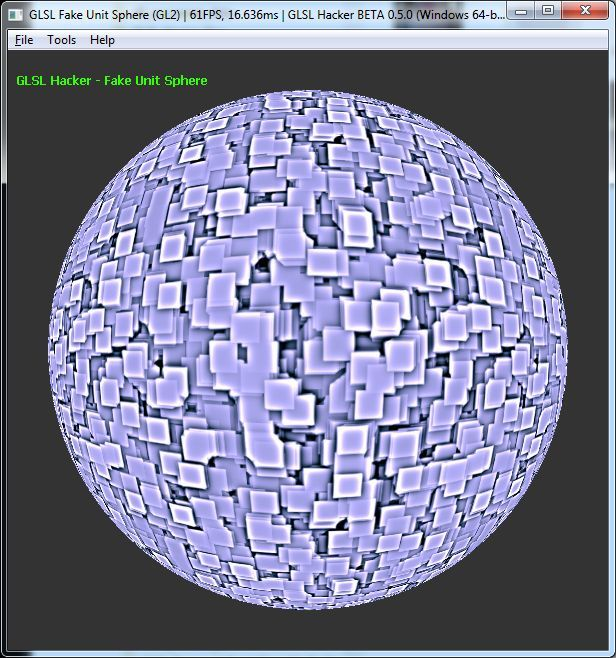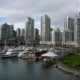# Circle, Disc and Fake Sphere in GLSL (Shader Library)

Article index:

## 4 – A Fake Sphere in GLSLThe fake sphere is a simple port of this GeeXLab demo.

```#version 120
void main()
{
gl_Position = gl_ModelViewProjectionMatrix * gl_Vertex;
gl_TexCoord = gl_MultiTexCoord0;
}
```

```#version 120
uniform sampler2D tex0;
uniform vec4 bkg_color;
uniform float time;
void main (void)
{
vec2 p = -1.0 + 2.0 * gl_TexCoord.xy;
float r = sqrt(dot(p,p));
if (r < 1.0)
{
vec2 uv;
float f = (1.0-sqrt(1.0-r))/(r);
uv.x = p.x*f + time;
uv.y = p.y*f + time;
gl_FragColor = texture2D(tex0,uv);
}
else
{
gl_FragColor = bkg_color;
}
}
```

## 2 thoughts on “Circle, Disc and Fake Sphere in GLSL (Shader Library)”

1.Nathan

You can anti-alias the fake sphere’s edges by modifying the alpha channel with a smoothstep function. The one used in the Disc demo should do the trick, just adjust the values so it won’t be so thick.

2.JeGX Post Author

Thanks Nathan. I quickly ported the fake sphere demo without thinking to the antialiasing and as you said, the smoothstep function can help to antialiase.